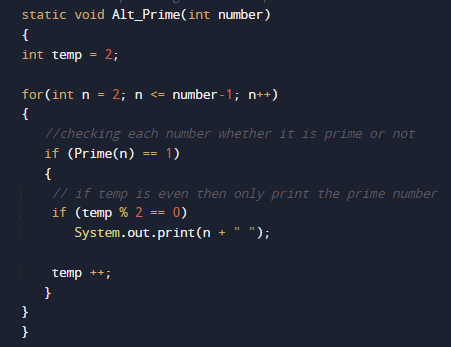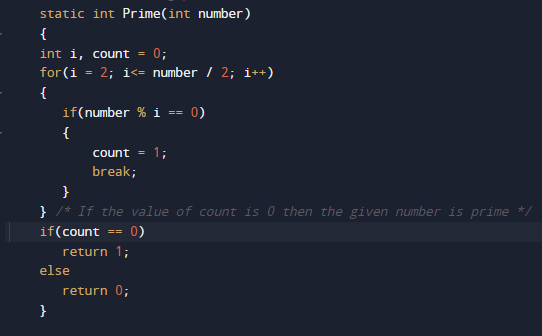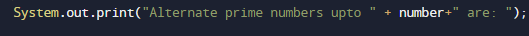# Java Program to display alternate prime numbers

In this tutorial you will learn about the Java Program to display alternate prime numbers and its application with practical example.

In this tutorial, we will learn to create a Java Program to display alternate prime numbers using Java programming.

## Prerequisites

Before starting with this tutorial we assume that you are best aware of the following Java programming topics:

• Java Operators.
• Basic Input and Output function in Java.
• Class and Object in Java.
• Basic Java programming.
• If-else statements in Java.
• For loop in Java.

## Prime Number:

A prime number is a number such that is either divides by 1 or itself(Number itself).

For example, 2, 3, 5, 7,11,13 and etc. are prime numbers.

## Java Program to display alternate prime numbers

In this program we would find alternate Prime number Between given number .first of we would take a value from user and find display alternate prime numbers, Let’s have a look at the code.

## Output

Alternate Prime number between given number areIn the above program, we have first declared and initialized a set variables required in the program.

• number= it will hold entered number.
• count =   it will count number is  prime or not.
• temp =  it print prime number with in the given range.
• n        = for iteration.

After that we take a number from user we will print prime number between the given range.now we will pass the given range to function Alt_Prime(number) where we find prime number between given range.with in the function we will call function named with Prime() where we calculate Prime numbers.This  function find Prime number within the range and returns true to Alt_Prime() function .where we print Prime numbers.

In this tutorial we have learn about the Java Program to display alternate prime numbers and its application with practical example. I hope you will like this tutorial.